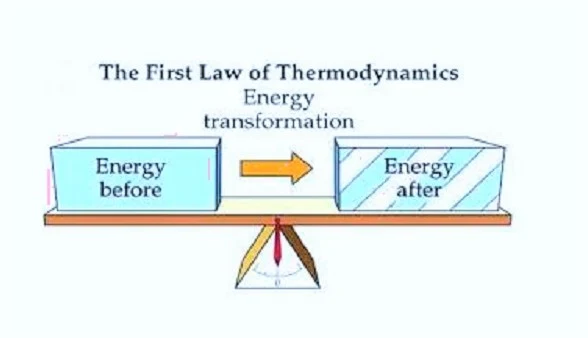# First Law of Thermodynamics || Second Law of Thermodynamics || Third Law of Thermodynamics || Kelvin Statement || Plank's Statement || Celsius Statement

### First Law of ThermodynamicsThe First law of Thermodynamics is the law of conservation of energy. It can be states as, " The energy can neither be created nor be distroyed it can only be converted from one form to another form".
The First law of Thermodynamics can be expalaind in the terms of 3 equations.

1. The amount of work done (dw) which is performed.
2. The amount of heat (dQ) which is transferd.
3. The internal energy or intrinsic (du) which is started in the system.
Thus, the first law of thermodynamics Mathmaticaly can be stated sa, " When the amount of heat be (dQ) is transfer to or by the system and work done on or by the system. Using some amount of heat and the rest heat this used for chenging the internal energy of the system.
Thus, it can be expressed as-
du = dQ - dw
or
dQ = du + dw
Note : When the system accept heat and work is done then the sign of dQ is positive. When the system reject heat and work is done then the sign of dQ is negative.

### Second Law of Thermodynamics

The first law of thermodynamics does not tells that the conversion of heat into mechanical works governing this property an other law comes which is known as the second law of thermodynamics.

### Kelvin Statement :

Acording to Kelvin statement it is impossible to continuous supply of work from a body by cooling in it to a lower temprature that of its surrounding.

### Plank's Statement :

According to this statement it is impossible for the plank to continue the engine working in full cycle which produces no effect other than pricing the heat and cooling of the reservious.

### Celsius Statement :

It is impossible to create an engine which can heat the heat from low temperature to high temperature without doing any external work, according to the statement of Salius.

### Other Definition of Second Law of Thermodynamics :

It is impossible for self-acting equipment working in a cyclic process without transferring any external energy from low temperature to high temperature.